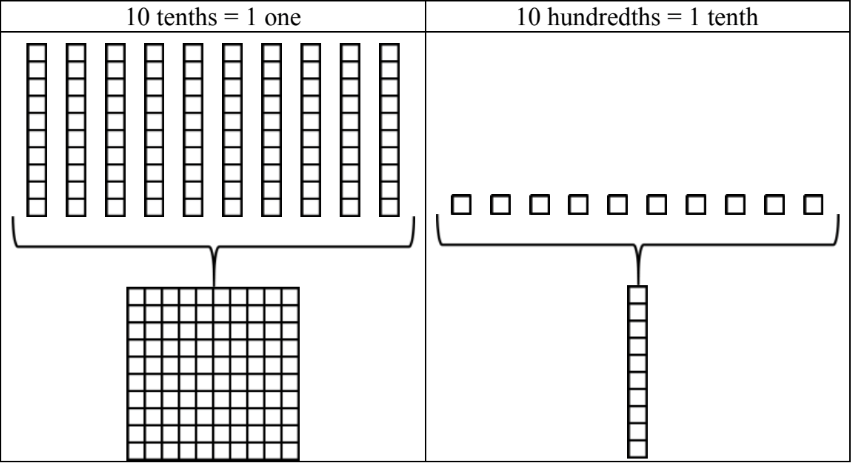#### All in One Place

Everything you need for better grades in university, high school and elementary.#### Learn with Ease

Made in Canada with help for all provincial curriculums, so you can study in confidence.#### Instant and Unlimited Help

0/1
##### Intros
###### Lessons
How to add decimals with base ten (block) models
0/14
##### Examples
###### Lessons
1. Regrouping practice for decimals with block models
Regroup so that each place value only has one digit. Use base ten (block) models to help.
1. 17 tenths = ____ ones + ____ tenths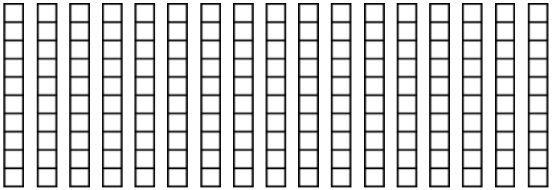2. 4 ones + 12 tenths + 39 hundredths = ____ ones + ____ tenths + ____ hundredths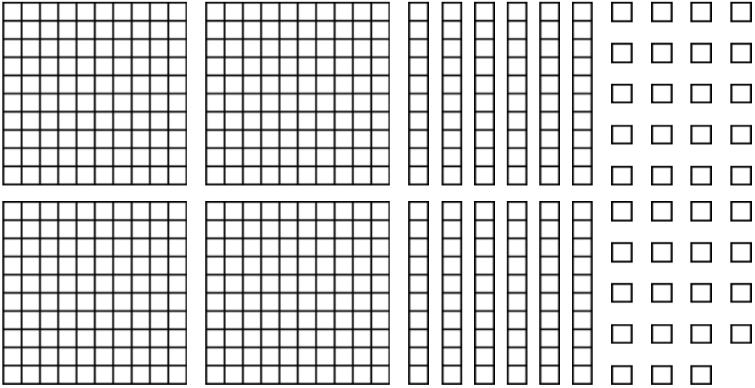3. 6 ones + 29 tenths + 16 hundredths = ____ ones + ____ tenths + ____ hundredths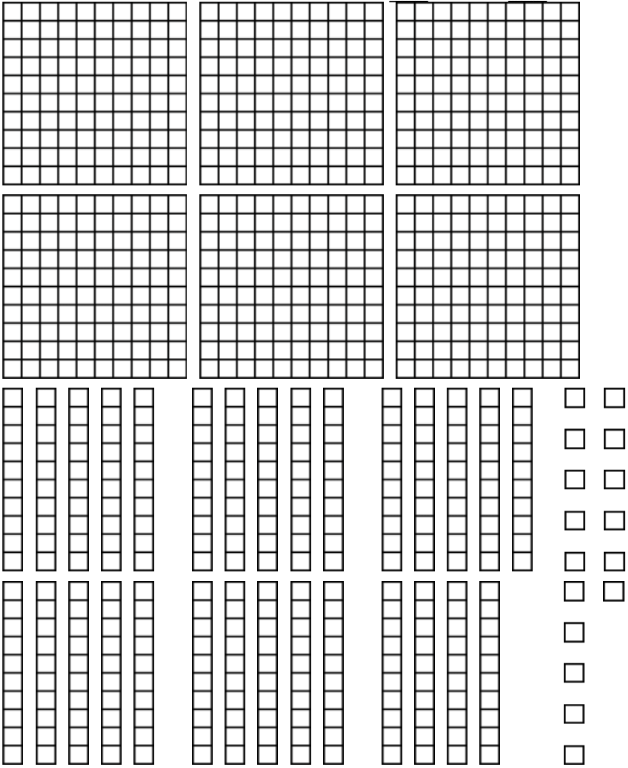1.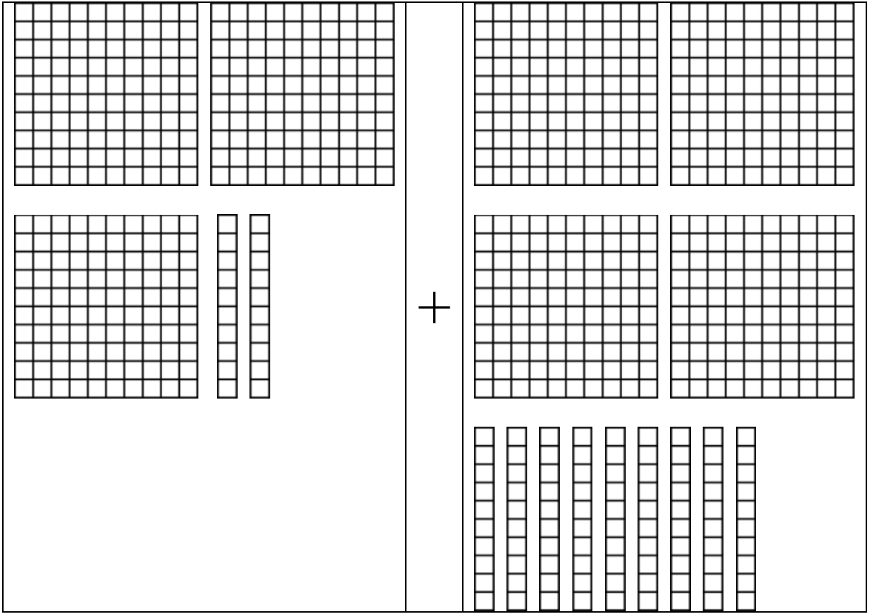2.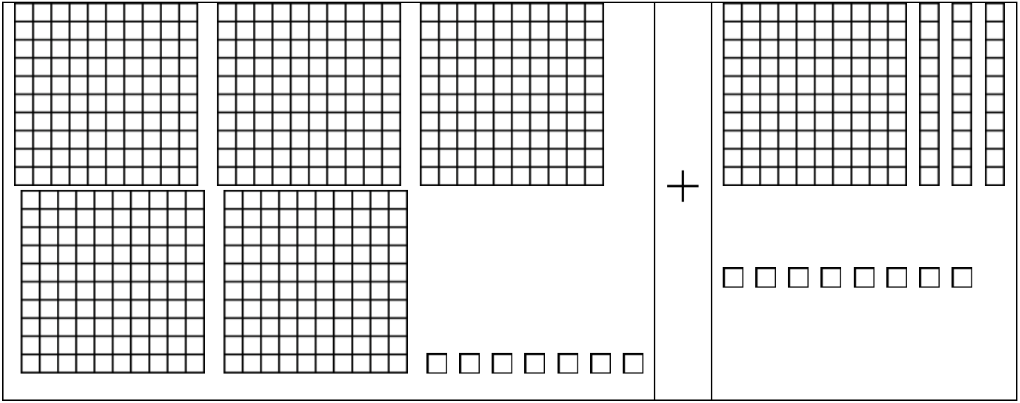3.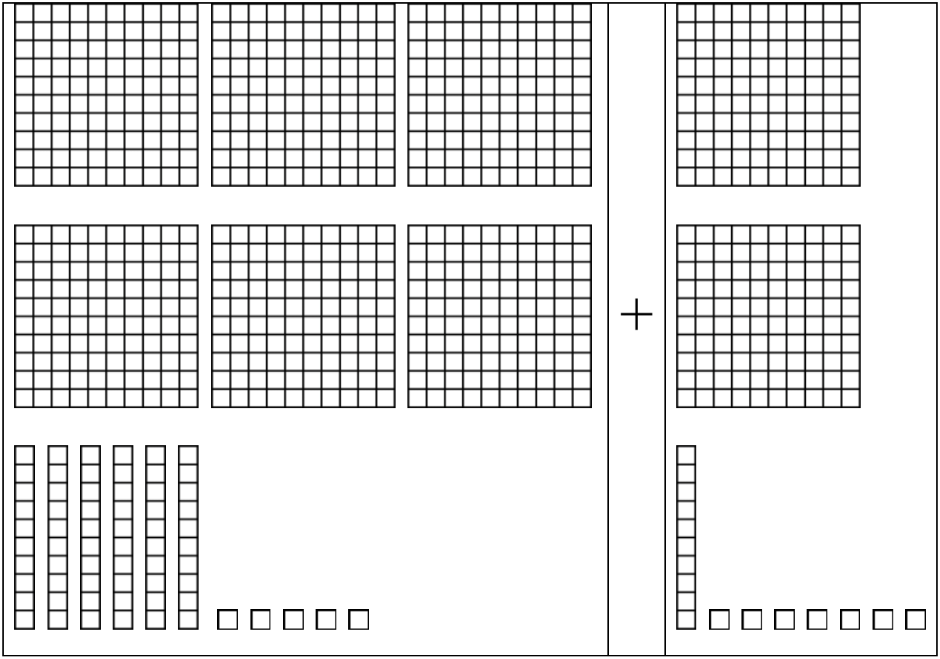3. Adding decimals on a grid
Add the decimals by lining up on the grid. Regroup where necessary.
1. 34.8 + 5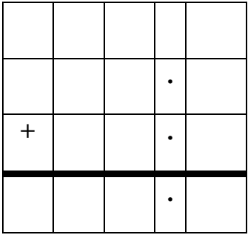2. 4.6 + 2.9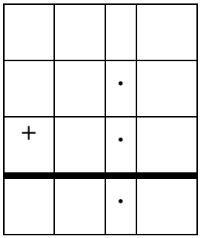3. 41.03 + 5.72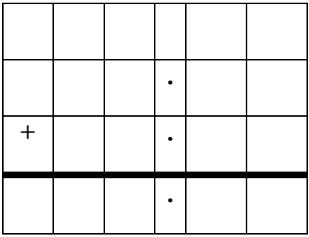4. 35.84 + 6.5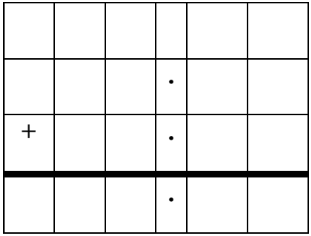4. Adding decimals and rounding decimal sums
1. 7.6 + 2.14
2. 80.65 + 19.23
3. 18.24 + 3.17
4. 98 + 38.97
0%
##### Practice
###### Topic Notes

In this lesson, we will learn:

• How to add decimal numbers using base ten (block) models
• That adding decimal numbers is the same as adding whole numbers, which involves lining up the right place values and regrouping

Notes:

• To add decimals with base ten (block) models:
• Remember the three types of blocks used to represent the ones, tenths, and hundredths place values
• "One whole" or hundred block = ones place value
• Column (stick or rod) = tenths place value
• Single square = hundredths place value